# Kerala Syllabus 7th Standard Maths Solutions Chapter 14 Pie Charts

## Kerala State Syllabus 7th Standard Maths Solutions Chapter 14 Pie Charts

### Pie Charts Text Book Questions and Answers

Pie charts Textbook Page No. 188

Pie charts are usually drawn to show a single numerical fact split into categories and to makes comparisons between these.
Such a picture is drawn with the size of each part depending on the number it represents.

Food for thought

Pie is the name of a baked dish very much popular in England and USA.It is divided and shared by cutting into slices as shown in the picture. The name pie chart originates from this.Bar and Circle Textbook Page No. 190

The household expenses of Renu’s family is shown as a bar graph and as a pie-chart below: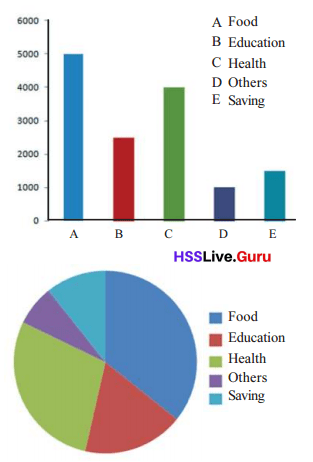Look at the bar graph. We can easily see the amounts spent under each head and compare one another, but we cannot directly see what fraction of the total each is. That is easier to see in the pie chart. But the actual amount of each is not easy to see in it. Thus, each type of picture has certain advantages and defects.

Let’s make a table

All students of Class 7 in a school are in one of the various clubs. A pie chart showing the number of students in each club is shown below.There are 50 children in Vidyarangam. Make a table showing the number of children in each club.
Given data:
Number of children in vidyarangam=50
Solution:
From the pie chart, we can observe that science club is half of the vidyarangam club. Therefore, number of children in science club will be $$\frac{1}{2}$$×50=25.
Number of children in social and english club seems to be equal to the science club. Therefore, number of children in social and english club will be 25.
Number of children in maths club are three times of the science club. Therefore, number of children in maths club will be 3×25=75.
From our assumptions, we can make the below table.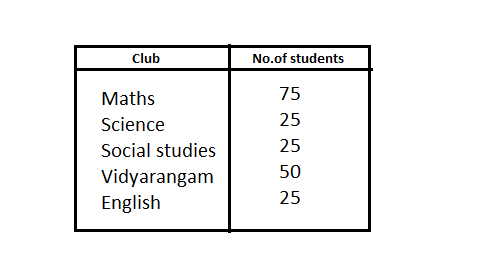Piechart with computers Textbook Page No. 192

Pie charts can be easily drawn using a computer.

Open Libre Office Calc program and type in the details as below: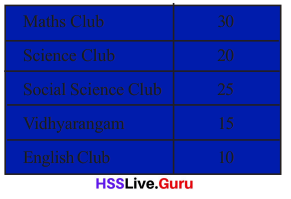Click on any cell and choose Insert → chart → pie. This makes a pie-chart. Change the numbers and see what happens to the picture.
Given data:
From the above table, we get the number of students from each club and the total number of students belonging to all the clubs.
Total number of students: 30+20+25+15+10=100
To draw a pie chart, we need to calculate the angle of each sector.
Formula:
Angle of sector = 360º×$$\frac{Given value}{Total sum of all the values}$$
Solution:
Angle of sector for maths club is calculated as 360º×$$\frac{30}{100}$$ = 108º
Angle of sector for science club is calculated as 360º× $$\frac{20}{100}$$ = 72º
Angle of sector for social science club is calculated as 360º× $$\frac{25}{100}$$ = 90º
Angle of sector for vidhyarangam club is calculated as 360º×$$\frac{15}{100}$$ = 54º
Angle of sector for english club is calculated as 360º×$$\frac{10}{100}$$ = 36º
Steps of Construction:
Step 1: Draw a circle with any convenient radius and mark the center as ‘O’.
Step 2: Next, mark a point A anywhere on the circumference of the circle and join OA.
Step 3: To represent maths club sector, construct ∟AOB=108º.
Step 4: To represent science club sector, construct ∟BOC=72º.
Step 5: To represent social science club sector, construct ∟COD=90º.
Step 6: To represent vidyarangam club sector, construct ∟DOE=54º.
Step 7: To represent english club sector, construct ∟EOA=36º.Electricity

The pie chart below shows the details of electricity supplied by the Kerala State Electricity Board during 2011-2012.What all facts do we get from this?
The above pie chart depicts supplied electricity in different sectors.
From the given pie chart, it is clearly observed that the electricity supplied to Household sector is more as it covers the major part when compared to others.
Industrial sector is on second place, Commercial on third place, other sectors on fourth place and agriculture sector consumes very less than the remaining sectors.
Conclusion:
From the observation, more electricity is supplied to household sector and while very less is supplied to agriculture sector.
The pie chart given beLow shows the revenue of Kerala State Electricity Board, during 2011-2012.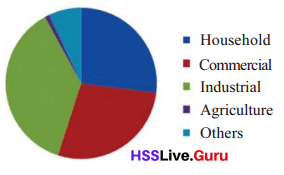What all information do we get from this?
From the observation, we can say that the revenue generated from Industrial sector is more and agriculture sector is very less.
Compare the two charts.
By comparing the above two pie charts,
We can observe that more electricity is supplied to household while most of the revenue is generated from industrial sector.
Approximately, same revenue is generated from other groups.
The electricity consumed and revenue generated by agriculture sector is less than all the other sectors.

Time management Textbook Page No. 194

Given below is the pie-chart showing how Aravind, a class 7 student spends his time:The circle is divided into 24 parts, each part indicating one hour.
What each colour shows is given below.Draw a bar graph showing these details.
Given that circle is divided into 24 parts representing 5 different groups. By observing the above pie chart, we can calculate the number of parts covered under each sector.
Step 1: Number of parts covered under school are 6.
Step 2: Number of parts covered under sleep are 8.
Step 3: Number of parts covered under studies are 4.
Step 4: Number of parts covered under play/exercise are 3.
Step 5: Number of parts covered under other activities are 3.Change to circle

The bar graph below shows the number of girls in the three divisions of Class 7 in a school.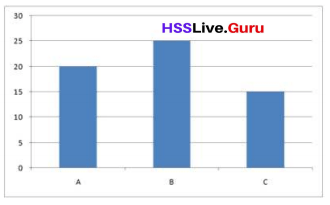Draw a pie chart of this.
To draw a pie chart, we need to calculate the angles for each sector. To find the angles of each division we can use the below mentioned formula.
Formula:
Angle of sector = 360º×$$\frac{Given value}{Total sum of all the values}$$
Given data:
The vertical line or y-axes represents the number count of the girls in each division and horizontal line or x-axes represents the division for which it belongs to.
Division A is marked completely till 20, so 20 girls fall under it.
Similarly, 25 girls belong to division B and 15 girls belong to division.
Total number of girls will be 20+25+15=60
Solution:
Division A: Angle of sector for A will be 360º× $$\frac{20}{60}$$=120º.
Division B: Angle of sector for B will be 360º× $$\frac{25}{60}$$=150º.
Division C: Angle of sector for C will be 360º× $$\frac{15}{60}$$=90º.
Steps of Construction:
Step 1: Draw a circle with any convenient radius and mark the center as ‘O’.
Step 2: Next, mark a point A anywhere on the circumference of the circle and join OA.
Step 3: To represent division A, construct ∟AOB=120º.
Step 4: To represent division B, construct ∟BOC=150º.
Step 5: To represent division C, construct ∟COA=90º.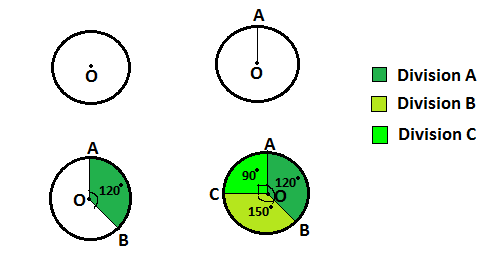Election Textbook Page No. 188

The picture below shown the votes each candidate got in a school election.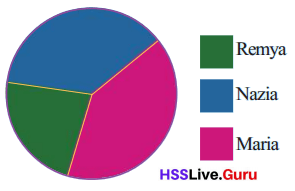• Who won the election?
By observing the pie chart, we can say that Maria won the election as major part of the votes are covered under her sector.

• What all information do you get from this picture?
From the picture, we can rank the candidates by observing the part covered under each of them.Maria ranks first and thus wons the election. Nazia is on the second place as the second largest number of votes are covered under her and Remya is on the third place, as she got less number of votes when compared to the other two.

House expenses

Look at this picture showing various expenses at Fathima’s home: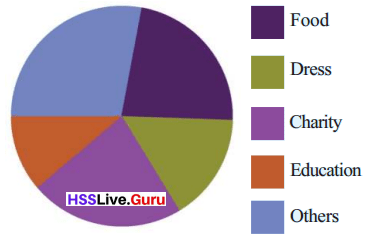For what, is the most amount spent?
By observing the pie chart, we can say that major amount was spent on other activities as the major part of the pie is covered under  it.
And the least?
Least amount was spent on the education sector as the least part was covered under it.
For what all are the same amount spent?
Same amount was spent on charity and food as both covers the equal part of pie.
How do we know that the same amount is spent on these?
We can know the areas where same amount is spent by calculating the angle of sector which can be known by using the below formula:
360º×$$\frac{Given value}{Total sum of all the values}$$
After calculating the sector angles/values, if any of the sector angle is same as other than we can say that same amount was spent on those groups.
What more do we get from the pictures?
We can get information such as,
Easy calculation of expenses made for different groups by using the above mentioned formula.
Easy understanding of major and minor expense made on groups by observing the pie chart.
We can get the order of the groups expenditure which is helpful to sort and plan the expenses accordingly.

A picture like this, which display information as parts of a circle is called pie chart.

Occupation

The pie chart below shows the various occupations of people in a panchayath.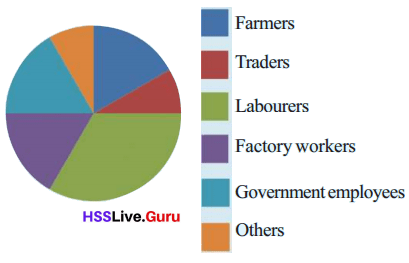• What is the occupation of the most number of people?
Labourers are the most number of people as the major part of circle is occupied by these group of people.

• Roughly, how many times the number of farmers is the number of labourers?
By observing the areas covered under farmers and labourers, we can assume that the number of labourers are double the number of farmers.

• Roughly what fraction of the total is the number of factory workers?
Let us make an assumption that the pie chart is divided into 6 equal sectors, labourers almost occupies 2 parts among the total parts.Therefore,we can assume that two-sixth of the complete circle is occupied by them.

• Arrange the occupations in the order of the number of people in each.
By observing the pie chart, the increasing order of working people will be others,traders,framers ,government employees,factory workers and labourers.

Make some more questions relating to this picture.
Which of the occupation has less number of people?
Which of the occupations has same number of people?
How can we say that the occupations are having the same number of people?Agriculture Textbook Page No. 190

The pie chart below shows how the total farmland in a panchayat is used for various crops.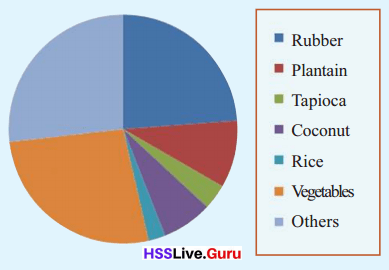Based on this figure, answer the following questions.

• For which is the least land used?
By observing the figure, we can say that rice occupies least land area when compared to the remaining crops as the part of area covered under it is less than the remaining.

• For which is the most land used?
By observing the figure, we can assume that the most of the land is used for other crops and vegetables as the major portion of the circle is covered under it.

• Roughly, what fraction of the total land is used for vegetables?
Let us make an assumption that the circle is divided into four major sectors. First sector represents the rubber crop, while second sector represents Plantain, Tapioca, Coconut and Rice.Third sector represents vegetables and fourth other crops.
Therefore, we can assume that one-fourth of the land is utilized for vegetables.

Let’s draw!

It was decided to have a vegetable garden in the school. Amaranth in half the plot and beans and brinjal in equal
parts of the other half. Let’s draw a pie chart showing this;
First draw a circle.
Half the plot is for amaranth.

How do we show this in our picture?
360º×$$\frac{1}{2}$$=180º, which is half of the complete circle.
How do we mark half the circle?
Draw a circle with any convenient radius and mark the center as ‘O’.
To mark half of the circle, construct ∟AOB=180º.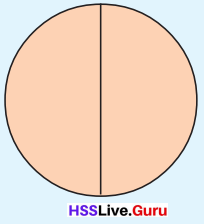Now to show the parts for beans and brinjal one the circle must be halved again.
Can’t you do it?
Given that:
Beans and brinjal in equal parts of the other half which is 180º×$$\frac{1}{2}$$=90º
Also colour each part to tell these apart.
To mark beans and brinjal in the circle, construct ∟BOC=90º AND ∟COA=90º.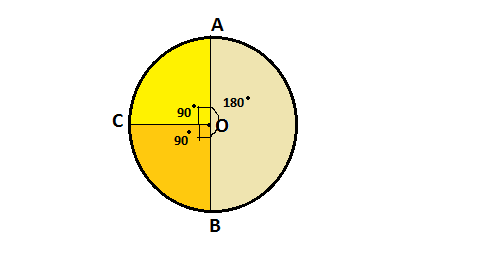Travel math Textbook Page No. 191

There are 40 students in class 7 A of a school. 20 of them come in the school bus, 5 of them walk and 5 ride bicycles
to school.
Let’s draw a pie chart showing these.
What fraction of children take the school bus?
Fraction of students who take school bus will be $$\frac{No.of students who prefer school bus}{Total students}$$=$$\frac{20}{40}$$.So, $$\frac{1}{2}$$ of total students take school bus.
This we can mark in a circle as before.
What fraction of children ride bicycles?
The number of students who ride bicycles will be $$\frac{5}{40}$$=$$\frac{1}{8}$$
How do we mark $$\frac{1}{8}$$ of the circle?
What angle should we draw for this?
$$\frac{1}{8}$$ of 360° = 45°The remaining part of the circle shows the number of children who walk to school.
• What fraction of the circle is this?
Given that 20 of them come to school by school bus,5 of them by bicycle and 5 of them by walk and the total number of students in 7A class are 40.
Solution:
Students who come by walk will be the difference of total students from students coming from other modes.So,the total number of students who come by walk will be 40-(20+5)=15
Fraction of the students who come by walk will be $$\frac{15}{40}$$=$$\frac{3}{8}$$
So, $$\frac{3}{8}$$ of the total students come by walk.

• How much degrees is its angle?
Angle of sector for walk will be 360º×$$\frac{3}{8}$$=135º.

School clubs

In a school, there are 100 students in Class 7 and each one is a member of some club.
The table shows the number of students in various clubs.We want to draw a pie chart of this.
What all fractions of the circle should we mark to show the number of members of various clubs?
There are loo students altogether.
Math club has 30 numbers.
To show this, we must mark $$\frac{30}{100}$$ of the circle.
What angle should we draw to get this?
360° × $$\frac{30}{100}$$ = 108°
Similarly, what are the angles we have to draw to show the numbers in other clubs?
Science : 360° × $$\frac{20}{100}$$ = 72°
Social Science : 360° × $$\frac{25}{100}$$ = 90º
English : 360° × $$\frac{10}{100}$$ = 36º
Vidhyarangam : 360° × $$\frac{15}{100}$$ = 54º
Now we can draw the pie chart:Grade chart Textbook Page No. 193

In Class 7 ofa school, 25% got A grade, 45% got B, 20% got C and the remaining D. Let’s draw a pie chart of this.
Let’s compute the fractions of circles to show those who got different grades:
We must use 25% of the circle to show this.
360 × $$\frac{25}{100}$$ = 90°

40 % got B, and the angle is
= 360° × $$\frac{45}{100}$$ = 162°
Can’t you compute the angles to be drawn to show those who got C and D grades, and draw the pie chart?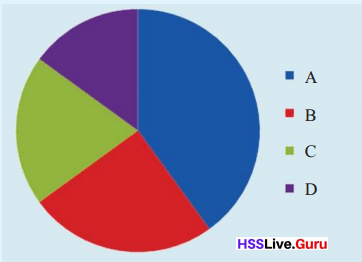Collect such information about your class and other classes in your school and make pie charts. Display these in Maths lab.
20% got C, the angle is 360° × $$\frac{20}{100}$$=72º.
Given that 25% got A grade, 45% got B, 20% got C.
Let us assume 100 as the total percentage, so the percentage D got will be 100-(25+45+20)=10%.
So, the angle will be 360° × $$\frac{10}{100}$$=36º

Now draw pie charts of the following

• The final match of the school cricket tournament was between Ramanujan House and C.V. Rarnan House. The details of the scores are given below. Draw a pie chart of each: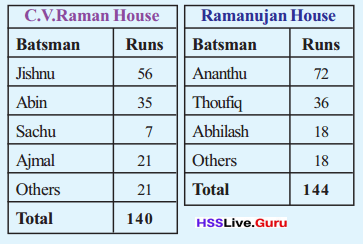Given table has the data of the school cricket tournament between Ramanujan House and C.V. Rarnan House.
Formula used to find the angle of sector made by each player:
360º×$$\frac{Given value}{Total sum of all the values}$$

C.V.Raman House:
Solution:
Runs made by Jishnu will be 360º× $$\frac{56}{140}$$=144º
Runs made by Abhi will be 360º× $$\frac{35}{140}$$=90º
Runs made by Sachu will be 360º× $$\frac{7}{140}$$=18º
Runs made by Ajmal will be 360º× $$\frac{21}{140}$$=54º
Runs made by other players will be 360º× $$\frac{21}{140}$$=54º
Steps of Construction:
Step 1: Draw a circle with any convenient radius and mark the center as ‘O’.
Step 2: Next, mark a point A anywhere on the circumference of the circle and join OA.
Step 3: To represent the runs made by Jishnu, construct ∟AOB=144º.
Step 4: To represent the runs made by Abhi, construct ∟BOC=90º.
Step 5: To represent the runs made by Sachu, construct ∟COD=18º.
Step 6: To represent the runs made by Ajmal, construct ∟DOE=54º.
Step 7: To represent the runs made by other players, construct ∟EOA=54º.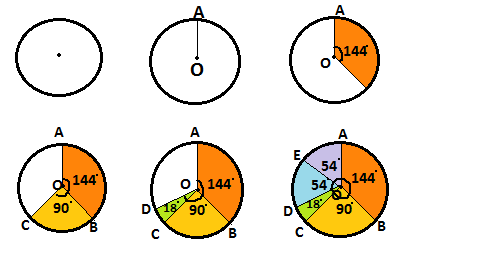Ramanujan House:
Solution:
Runs made by Ananthu will be 360º× $$\frac{72}{144}$$=180º
Runs made by Thoufiq will be 360º× $$\frac{36}{144}$$=90º
Runs made by Abhilash will be 360º× $$\frac{18}{144}$$=45º
Runs made by other players will be 360º× $$\frac{18}{144}$$=45º
Steps of Construction:
Step 1: Draw a circle with any convenient radius and mark the center as ‘O’.
Step 2: Next, mark a point A anywhere on the circumference of the circle and join OA.
Step 3: To represent the runs made by Ananthu, construct ∟AOB=180º.
Step 4: To represent the runs made by Thoufiq, construct ∟BOC=90º.
Step 5: To represent the runs made by Abhilash, construct ∟COD=45º.
Step 6: To represent the runs made by other players, construct ∟DOA=45º.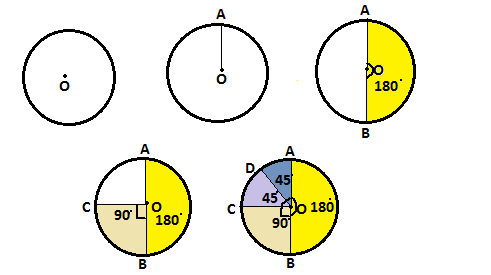• There are 1600 books in the school library, classified as shown below.
Short story – 320
Poetry – 192
Novel – 384
Science – 544
Biography – 160
Draw a pie chart showing this
Given data depicts different classifications of 1600 books in library.
Formula:
360º×$$\frac{Given value}{Total sum of all the values}$$
Solution:
Angle of sector for short story books will be 360º×$$\frac{320}{1600}$$=72º.
Angle of sector for poetry books will be 360º×$$\frac{192}{1600}$$=43.2º.
Angle of sector for novel books will be 360º×$$\frac{384}{1600}$$=86.4º.
Angle of sector for science books will be 360º×$$\frac{544}{1600}$$=122.4º.
Angle of sector for biography books will be 360º×$$\frac{160}{1600}$$=36º.
Steps of Construction:
Step 1: Draw a circle with any convenient radius and mark the center as ‘O’.
Step 2: Next, mark a point A anywhere on the circumference of the circle and join OA.
Step 3: To represent the short story classification, construct ∟AOB=72º.
Step 4: To represent the poetry classification, construct ∟BOC=43.2º.
Step 5: To represent the novel classification, construct ∟COD=86.4º.
Step 6: To represent the science classification, construct ∟DOE=122.4º.
Step 7: To represent the biography classification, construct ∟EOA=36º.In a survey, the number of students who like diffèrent kinds of books were found as follows.
Short story – 84
Poetry – 36
Novel – 48
Science – 60
Biography – 12
Given data depicts the number of students who likes different kinds of books.The total number of students are 240.
Formula:
360º×$$\frac{Given value}{Total sum of all the values}$$
Solution:
Angle of sector for short story books will be 360º×$$\frac{84}{240}$$=126º.
Angle of sector for poetry books will be 360º×$$\frac{36}{240}$$=54º.
Angle of sector for novel books will be 360º×$$\frac{48}{240}$$=72º.
Angle of sector for science books will be 360º×$$\frac{60}{1600}$$=90º.
Angle of sector for biography books will be 360º×$$\frac{12}{1600}$$=18º.
Steps of Construction:
Step 1: Draw a circle with any convenient radius and mark the center as ‘O’.
Step 2: Next, mark a point A anywhere on the circumference of the circle and join OA.
Step 3: To represent the number of students reading short story books, construct ∟AOB=126º.
Step 4: To represent the number of students reading poetry books, construct ∟BOC=54º.
Step 5: To represent the number of students reading novel books, construct ∟COD=72º.
Step 6: To represent the number of students reading science books, construct ∟DOE=90º.
Step 7: To represent the number of students reading biography books, construct ∟EOA=18º.Draw a pie chart of this also. Compare the two pie-charts.
Are books bought according to the preference of the students?
By comparing both the pie charts, we can say that the short story and poetry books are not according to the preference. Short story and poetry books are less while the number of students preferring those classification are more.
Number of students who prefer short story book are 126 while the available books are 72.
Number of students who prefer poetry book are 54 while the available books are approximately 43.

• Collect various pictographs, bar graph and pie charts from various periodicals. Analyse and compare these.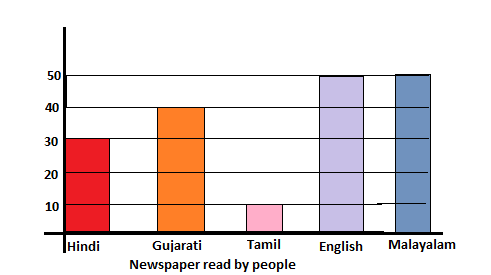The above bar graph represents the newspaper read by different people in a society.
From the graph, we can observe that
The number of people preferring Hindi newspaper are 30.
The number of people preferring Gujarathi newspaper are 40.
The number of people preferring Tamil newspaper are 10.
The number of people preferring English newspaper are 50.
The number of people preferring Malayalam newspaper are 50.
So, the total number of people reading all the newspaper are 180.
The same can be represented in pie chart as,
Hindi newspaper: 360º×$$\frac{30}{180}$$=60º
Gujarati newspaper: 360º×$$\frac{40}{180}$$=80º
Tamil newspaper: 360º×$$\frac{10}{180}$$=20º
English newspaper: 360º×$$\frac{50}{180}$$=100º
Malayalam newspaper: 360º×$$\frac{50}{180}$$=100º
So, the pie chart for the above table will be: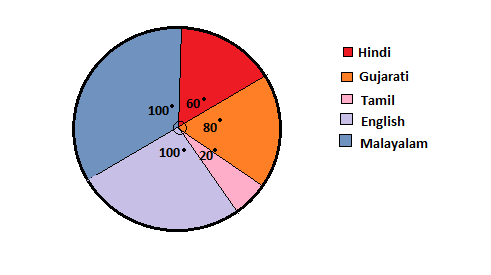By comparing both the charts
Both the data representation has its own advantage and disadvantages. Bar graph helps in reading the numeric data more easily by observing the vertical bar. While, the pie chart represents the proportions of each category by observing the slices.

• Make a pie chart of the number of sùidents in various classes of your school, using computer
Data collected:
Class 1: 55
Class 2: 45
Class 3: 60
Class 4: 57
Class 5: 63
Class 6: 50
Class 7: 80
Class 8: 60
Class 9: 80
Class 10: 50
Total number of students are 600.
Formula:
360º×$$\frac{Given value}{Total sum of all the values}$$
Solution:
Class 1: 360º×$$\frac{55}{600}$$=33º
Class 2: 360º×$$\frac{45}{600}$$=27º
Class 3: 360º×$$\frac{60}{600}$$=36º
Class 4: 360º×$$\frac{65}{600}$$=39º
Class 5: 360º×$$\frac{55}{600}$$=33º
Class 6: 360º×$$\frac{50}{600}$$=30º
Class 7: 360º×$$\frac{80}{600}$$=48º
Class 8: 360º×$$\frac{60}{600}$$=36º
Class 9: 360º×$$\frac{75}{600}$$=45º
Class 10: 360º×$$\frac{65}{600}$$=39º
Pie chart for the above calculated angles can be drawn as shown below.